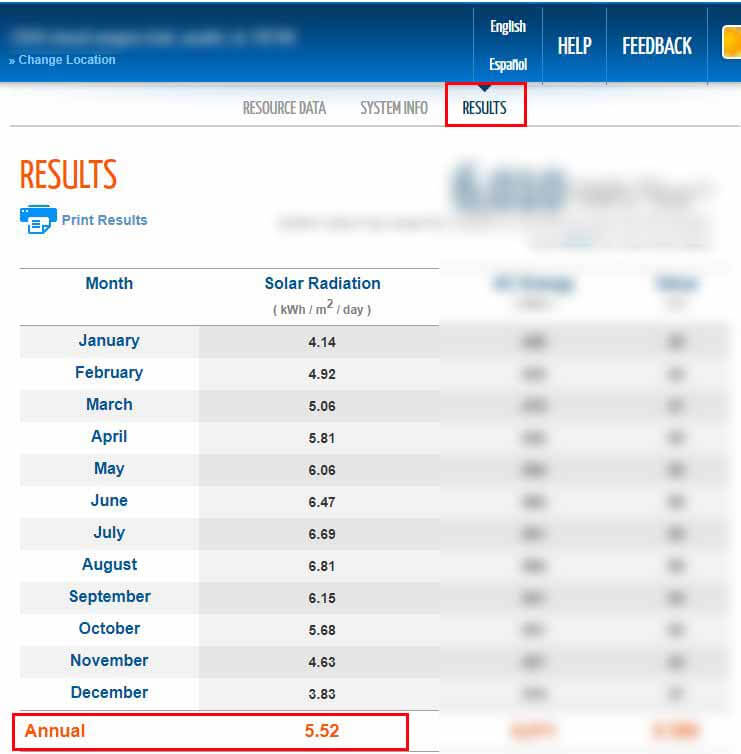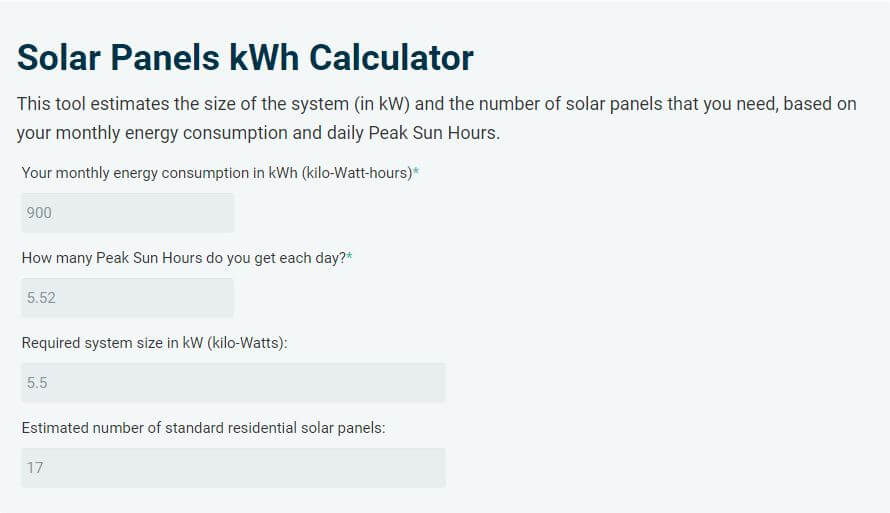# How many solar panels do I need to power my house? A 2 step guide to estimating how much solar power you need to run your house

You’re considering solar for your home, and you’re wondering “how many solar panels do I need?“.

However, since residential solar panels have power outputs ranging from 250 to 400 watts, you could have different numbers of solar panels for the same amount of solar power.

So, the first and more important question to ask is: how much solar power do I need?

To answer this question, you’ll need 2 pieces of information:

• Your monthly energy consumption (in kWh).
• An estimate of the amount of sunlight that is available to you.

In this article, I’ll show you how to determine these values and use them to estimate the amount of solar power needed to run your house.

## How many solar panels do I need to power my house?

According to the EIA, the average home in the U.S. consumes about 900 kWh of energy per month. A solar installation that could generate this amount of energy, could be rated at anywhere from 4.5 to 7.5 kW (kilo-Watts), depending mainly on where it is installed.

In terms of solar panels, this is equivalent to 14-24 residential solar panels (320W).

For example, in Austin, TX, you’d need around 5.5 kW of solar power to produce 900 kWh of energy per month. A system of this size would consist of 18 solar panels, rated at 320W each.

In any case, the size of the system and the number of solar panels that you need to power your house generally depend on 2 variables:

• Your monthly energy consumption in kWh.
• The amount of sunlight available in your location.

First things first.

### How much energy do you consume per month?

Energy consumption, and production for that matter, is measured in kWh, which stands for kilo-Watt-hours. This piece of information is generally available on your electricity bills.

For example, the following image is from an electricity bill that contains historical energy consumption in kWh:

Above is an example of both a table and a pole graph showing power consumption in kWh. The table shows monthly energy consumption values, while the graph displays the average daily consumption for each month.

In your case, the electricity bill will probably be different, but generally, it will contain enough data for you to figure out your average monthly energy consumption.

If you have enough data, calculate the sum of your energy usage from each of the past 12 months, and divide that by 12. This will result in your annual average monthly energy consumption in kWh.

Once you have an idea about your energy needs, the next step is to figure out how much sunlight is available to you.

### How much sunlight do you get?

The amount of sunlight that an area receives is referred to as Solar Irradiance and is measured in Watt per area. The conventional unit for this is W/m² (Watts per Square Meter).

Solar panels need exactly 1000W/m² of sunlight to produce their rated output. For example, a 320W residential solar panel can only produce 320 Watts of power at a given moment if it’s getting 1000W/m² of sunlight at that moment.

If the same 320W solar panel received 1000W/m² (Watts per square meter) of sunlight for one hour, it could be said to have received 1000Wh/m² (Watt-hours per square meter) of sunlight energy and generated 320Wh of energy by the end of that hour

If our solar panel received 500W/m² of sunlight for 4 hours, it could be said to have received 2000Wh/m² of sunlight energy and generated 640Wh of energy by the end of those 4 hours.

In other words, if you have an estimate of how much energy you’re getting from the sun (in Wh/m²), you can estimate how much energy a solar panel would produce, given that you know the rated output of that solar panel.

Conversely, if you know how much energy you need to produce, you can estimate the size of the system (in kW) needed to produce it.

So, how do you measure the average amount of energy you’re getting from the sun?

The answer is Peak Sun Hours.

#### What is a Peak Sun Hours?

Peak Sun Hours is a unit that measures the amount of energy that an area receives from the sun. 1 Peak Sun Hour is equivalent to 1000 Wh/m² (1 kWh/m²) of sunlight energy.

If a location received 700 W/m² of sunlight for 5 hours, it can be said to have received 3.5 Peak Sun Hours by the end of those 5 hours.

A location that – on average – receives 5000 Wh/m² per day (5 kWh/m²/day), can be said to receive 5 Peak Sun Hours per day.

For example, if we installed a 1 kW solar array in such a location, the installation would – on average – generate 5 kWh of energy per day:

Energy Production per day (kWh/day) = Rated Power (kW) x Peak Sun Hours/day

Energy Production per day (kWh/day) = 1 kW x 5 Peak Sun Hours/day

Energy Production per day (kWh/day) = 5 kWh/day

In the same location, if a person needs to produce 30 kWh of energy per day, they’d need 6 kW of solar power:

Rated Power (kW) = Energy Production per day (kWh/day) ÷ Peak Sun Hours/day

Rated Power (kW) = 30 kWh/day ÷ 5 Peak Sun Hours/day

Rated Power (kW) = 6 kW

#### How many peak sun hours do you get?

Estimating the average amount of energy that you get from the sun does not require technical knowledge or the use of fancy gadgets. Thanks to tools such as NREL’s PVWatts Calculator, all you need is an address.Once your address is submitted, under the “results” section, the tool will provide monthly and annual averages of the peak sun hours that you receive each day. What you need for these calculations is the annual average.

For example, I submitted an address in Austin, TX, and the tool estimates that – on average – this location receives 5.52 Peak Sun Hours per day:Peak Sun Hours differ from location to location, so make sure to submit the right address. The tool will do the rest.

### How much solar power do I need?

As mentioned above, if you know the amount of sunlight energy (Peak Sun Hours) that is available to you, and the amount of energy that you need to offset (kWh), you can estimate the size of the system that you need:

Rated Power (kW) = Energy Production per day (kWh/day) ÷ Peak Sun Hours/day

Or you can use this formula for your monthly energy production:

Rated Power (kW) = Monthly Energy Consumption (kWh/month) ÷ (Peak Sun Hours x 30)

To make things even easier, I’ve made the following calculator.

## Solar panels kWh calculator

Based on your energy needs and the Peak Sun Hours that you get, the following calculator estimates the size of the system and the number of solar panels that you need:

for example, I used 900 kWh as the monthly energy consumption and 5.52 as the Peak Sun Hours, here are the results:The calculator estimates that with this amount of average daily Peak Sun Hours, a person would need 5.5 kW of solar power to produce 900 kWh of energy per month.

Such a PV installation would consist of 17 solar panels, these solar panels are rated at 330W each.

Please note that there are other variables to take into consideration. Such as voltage drops, inverter losses, thermal losses, the occasional dust/dirt on the solar panels, etc… These losses can add up to 25% of the overall energy production. Generally, a professional solar installer will account for these losses.

Another important thing to note is that in some cases, there isn’t enough space to fit all the solar panels that you need. This can be due to roof orientation, surrounding trees, etc…

## Related topics: